# Oscillator using just a diode, C and R

#### ApacheKid

Joined Jan 12, 2015
1,094
Is this feasible? searching for it seems to keep finding stuff related to Gunn diodes, tunnel diodes and so forth.

Consider: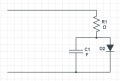If a suitable voltage is applied, then C1 will begin to charge through R1, D2 will have an insufficient voltage (less than around 0.6v) across it so will not conduct, no current will flow through, effectively and open circuit - all the while though, that voltage is rising.

As C1 charges, the voltage across D2 will rise until it reaches around 0.6v or so, then it will begin to conduct, at which point it begins to act as a discharge path for C1.

If C1 discharges to a sufficiently low voltage again, the voltage across D2 will fall below 0.6v and it will cease to conduct, so cease to provide a discharge path for C1.

So I'd expect C1 to again begin charging again and the entire cycle repeat itself.

On paper then it seems to offer the prospect of oscillation given suitable values for R and C.

Is this feasible? if not why not?

I'm not saying its useful, I'm sure it would have all kinds of drawbacks etc. but would it, could it, actually oscillate?

(I was just fiddling around with this (I used an LED that was lying around for the diode) and as I slowly increased the applied voltage, I did see what looked like huge instability and lots of high frequency noise on the scope (voltage across the diode) at certain points, but it was odd, not sure if it was oscillation or just noise due to other factors).

Last edited:

#### ZCochran98

Joined Jul 24, 2018
221
From a theory perspective, we can look at this a couple ways: using an ideal diode (i.e.: an open when the voltage is below the knee voltage, and a voltage drop when above the knee voltage), using a "first-approximation" of the diode (an open when the voltage is below the knee voltage and a resistor in series with a potential drop when above), or using the nonlinear model. Unfortunately, in all three cases, the system cannot oscillate without a way to disconnect the voltage source once the diode has "seen" a voltage sufficiently high-enough.

Let's take a look at the ideal case. In the ideal case, we would have a rise time of tau = RC, during which the capacitor voltage increases until it reaches (in this example) 0.6V, when the diode would suddenly switch on. If you had set the diode knee voltage to 0, then we could treat the diode as a short (but would also have shorted out the capacitor). With the diode now conducting, it would have a mostly-constant 0.6V drop across it, because it's acting like a potential source. Because the capacitor is in parallel to it, it shares the same potential, and will no longer increase or change. If you were to suddenly short the power supply, the capacitor would discharge from 0.6V down to 0. If you were to disconnect it, it would probably stay charged at slightly less than 0.6V (it would discharge through the diode just enough so that its voltage drops to just below the knee voltage, switching the diode off). If we're allowing for "perfectly ideal" diode with no resistance, then the capacitor might drop immediately to zero volts, but the current would be infinite (I = C*dV/dt).

In the "first-approximation" case, it's similar, except now the voltage across the capacitor will still rise, but at a significantly lower rate, because some of the current is redirected through the diode. The equation that models this particular behavior would be:
$RC\frac{dV_c(t)}{dt} + \left(1+\frac{R}{R_d}\right)V_c(t) = V_0 + \frac{R}{R_d}V_k$
Here, I'm using C to be capacitance, R to be resistance, V_k to be the knee voltage (0.6V in your example), V_c(t) to be the voltage across the capacitor, R_d to be the diode effective "on" resistance, and V_0 to be the supply voltage. This is a first-order equation with no forced oscillatory terms included, so the solution is an exponential:
$V_c(t) = k\mathrm{e}^{-\frac{R+R_d}{R_dRC}t} + \frac{RV_k + R_dV_0}{R_d+R}$
for some constant k that satisfies any initial conditions (in this case, you'd set V_c(0) = V_k = 0.6, as that's the voltage it would be when this equation becomes applicable). As you can see, there are no oscillatory terms here - just exponential ones.

Finally, the realistic nonlinear diode case has a similar result, but the equation of behavior, to put it mildly, is terrifying beyond all reason, and has no "general solution":
$RC\frac{dV_c(t)}{dt} + RI_s\left(\mathrm{e}^{\frac{eV_c(t)}{nk_BT}}-1\right) + V_c(t) = V_0$
I_s is the reverse current through the diode (very, very small), e is electron charge (not to be confused with Euler's number, e), k_B is the Boltzmann constant, T is temperature in Kelvin, and n is some constant related to silicon doping. The value "k_B*T/e" is approximately 26mV at room temperature. This equation is...awful, but will not cause oscillations, because its piecewise linear approximation (for small ranges of voltages across it) turns into the "first-approximation" case. The extreme cases also will not cause oscillation.

Now, your idea for a diode-based oscillator isn't terribly off the mark - they're just much more complicated than this, usually (or require specialized types of diodes with an inductor included in the design to incorporate a second-order factor into the equations of behavior). Take a look at Figs. 13 and 14 at this link to see something that works, very roughly, on the principle you were asking about. It uses a comparator that detects when the capacitor has charged and allows the capacitor to discharge through one of the diodes once it's reached a specific level.

As @nsaspook stated as well, you'd need a negative resistance to get single-port device oscillation as well - something that, the higher the voltage gets, the lower the current gets. That's where the specialized diodes (Gunn or tunneling diodes) come into play - they frequently have this negative resistance region of operation; the system they're in just needs tuned to it. The standard diode (LED or a normal PN-junction diode) does not exhibit this behavior (as far as I know, anyway).

Without seeing the output myself from your LED voltage, it's possible the output you were seeing with an LED was indeed noise, especially thermal noise. When diodes get hot, their behavior changes, possibly significantly. This can introduce noise (avalanche and shot(?) noise) and briefly change conductivity. In fact, some internal temperature sensors use diodes and their thermal properties to calculate the temperature inside a device without having to rely on an actual thermocouple.

#### BobTPH

Joined Jun 5, 2013
6,080
First, let’s analyze this as if your characterization of the diode is correct, that ot conducts nothing until its voltage reaches 0.6V. If this were the case, it would then conduct all the current coming through the resistor and the charge on the capacitor would remain the same, no oscillation.

But that is not the case, the diode conducts significant current at 0.5V and conducts far more current at 0.7V than it does at 0.6V. In this case, the real case, the voltage rises until the current it conducts drops exactly enough current to drop the rest of the input voltage across the resistor. Again, a steady state is reached with no oscillation.

Now, your homework assignment: look up the neon bulb oscillator, and tell us why that one is different and actually oscillates.

Bob

#### sparky 1

Joined Nov 3, 2018
732
1.) UJT structural model, 2.) an equivalent model, 3.) an improved equivalent model. The improved UJT model shown link below in yellow.
I do agree with the post above. tunnel diodes relaxation oscillators negative resistance have a field.
https://electronicsarea.com/unijunction-transistor-ujt/

I can understand how this can be confusing. I try to use a stable working oscillator circuits that can be reproduced easily also what is available is the programmable unijunction PUT 2n2646.
I recall having the same question about being just a diode. At one time UJT oscillators were very common waveform spike or sawtooth
example below 5.9k and 4nF giving 50khz waveform is spike.Last edited:

#### KeithWalker

Joined Jul 10, 2017
2,620
If you turn the diode around so that the cathode is connected to +, If the supply exceeds the reverse breakdown voltage of the diode, the circuit will become a relaxation oscillator as long as the resistor is high enough to limit the current to a safe value.

#### Ramussons

Joined May 3, 2013
1,321
1.) UJT structural model, 2.) an equivalent model, 3.) an improved equivalent model. shown in yellow link below.
I do agree with the post above. tunnel diodes relaxation oscillators negative resistance have a field.
https://electronicsarea.com/unijunction-transistor-ujt/

I can understand how this can be confusing. I try to use a stable working oscillator circuits that can be reproduced easily.
I recall having the same question about being just a diode. At one time UJT oscillators were very common.
View attachment 229975
Is'nt the UJT B1 and B2 interchanged? Supposed to be like this.

?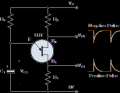#### sparky 1

Joined Nov 3, 2018
732
We used these as clocks, used potentiometer, the negative pulse followed by an inverting gate was an improvement.
One emitter two bases interchangeable. Good point that it is better to show both B1 and B2 outputs.
I dug out this old circuit for an arduino last year, I think the raspberry pi pico would be able to count pulses and produce
most any waveform a huge step forward again.

Last edited:

#### DickCappels

Joined Aug 21, 2008
9,513

#### ApacheKid

Joined Jan 12, 2015
1,094
From a theory perspective, we can look at this a couple ways: using an ideal diode (i.e.: an open when the voltage is below the knee voltage, and a voltage drop when above the knee voltage), using a "first-approximation" of the diode (an open when the voltage is below the knee voltage and a resistor in series with a potential drop when above), or using the nonlinear model. Unfortunately, in all three cases, the system cannot oscillate without a way to disconnect the voltage source once the diode has "seen" a voltage sufficiently high-enough.

Let's take a look at the ideal case. In the ideal case, we would have a rise time of tau = RC, during which the capacitor voltage increases until it reaches (in this example) 0.6V, when the diode would suddenly switch on. If you had set the diode knee voltage to 0, then we could treat the diode as a short (but would also have shorted out the capacitor). With the diode now conducting, it would have a mostly-constant 0.6V drop across it, because it's acting like a potential source. Because the capacitor is in parallel to it, it shares the same potential, and will no longer increase or change. If you were to suddenly short the power supply, the capacitor would discharge from 0.6V down to 0. If you were to disconnect it, it would probably stay charged at slightly less than 0.6V (it would discharge through the diode just enough so that its voltage drops to just below the knee voltage, switching the diode off). If we're allowing for "perfectly ideal" diode with no resistance, then the capacitor might drop immediately to zero volts, but the current would be infinite (I = C*dV/dt).

In the "first-approximation" case, it's similar, except now the voltage across the capacitor will still rise, but at a significantly lower rate, because some of the current is redirected through the diode. The equation that models this particular behavior would be:
$RC\frac{dV_c(t)}{dt} + \left(1+\frac{R}{R_d}\right)V_c(t) = V_0 + \frac{R}{R_d}V_k$
Here, I'm using C to be capacitance, R to be resistance, V_k to be the knee voltage (0.6V in your example), V_c(t) to be the voltage across the capacitor, R_d to be the diode effective "on" resistance, and V_0 to be the supply voltage. This is a first-order equation with no forced oscillatory terms included, so the solution is an exponential:
$V_c(t) = k\mathrm{e}^{-\frac{R+R_d}{R_dRC}t} + \frac{RV_k + R_dV_0}{R_d+R}$
for some constant k that satisfies any initial conditions (in this case, you'd set V_c(0) = V_k = 0.6, as that's the voltage it would be when this equation becomes applicable). As you can see, there are no oscillatory terms here - just exponential ones.

Finally, the realistic nonlinear diode case has a similar result, but the equation of behavior, to put it mildly, is terrifying beyond all reason, and has no "general solution":
$RC\frac{dV_c(t)}{dt} + RI_s\left(\mathrm{e}^{\frac{eV_c(t)}{nk_BT}}-1\right) + V_c(t) = V_0$
I_s is the reverse current through the diode (very, very small), e is electron charge (not to be confused with Euler's number, e), k_B is the Boltzmann constant, T is temperature in Kelvin, and n is some constant related to silicon doping. The value "k_B*T/e" is approximately 26mV at room temperature. This equation is...awful, but will not cause oscillations, because its piecewise linear approximation (for small ranges of voltages across it) turns into the "first-approximation" case. The extreme cases also will not cause oscillation.

Now, your idea for a diode-based oscillator isn't terribly off the mark - they're just much more complicated than this, usually (or require specialized types of diodes with an inductor included in the design to incorporate a second-order factor into the equations of behavior). Take a look at Figs. 13 and 14 at this link to see something that works, very roughly, on the principle you were asking about. It uses a comparator that detects when the capacitor has charged and allows the capacitor to discharge through one of the diodes once it's reached a specific level.

As @nsaspook stated as well, you'd need a negative resistance to get single-port device oscillation as well - something that, the higher the voltage gets, the lower the current gets. That's where the specialized diodes (Gunn or tunneling diodes) come into play - they frequently have this negative resistance region of operation; the system they're in just needs tuned to it. The standard diode (LED or a normal PN-junction diode) does not exhibit this behavior (as far as I know, anyway).

Without seeing the output myself from your LED voltage, it's possible the output you were seeing with an LED was indeed noise, especially thermal noise. When diodes get hot, their behavior changes, possibly significantly. This can introduce noise (avalanche and shot(?) noise) and briefly change conductivity. In fact, some internal temperature sensors use diodes and their thermal properties to calculate the temperature inside a device without having to rely on an actual thermocouple.

This analysis is very interesting, its been years since I did anything with differential equations but I'm fascinated when you say "there are no oscillatory terms". I take that to mean there are no periodic functions in the solution to Eq 1?

I also never knew we could embed math expressions in our posts, clearly there's a special markup dedicated to this!

The fact that you can predict it will not oscillate purely by mathematics is very impressive.

The third equation, the one with no general solution - is it conceivable that some solutions might include a periodic function?

Last edited:

#### BobTPH

Joined Jun 5, 2013
6,080
Of course, we have all bemoaned the fact that when we build an amplifier, it oscillates, and when we build an oscillator, it doesn’t.

Bob

•SamR and ApacheKid

#### ApacheKid

Joined Jan 12, 2015
1,094
Thanks guys, this is very interesting and although somewhat basic for most of you I am rusty, I studied electronics to some depth in the late 70s early 80s but my knowledge has become very fragmented over time!

Last edited:

#### ZCochran98

Joined Jul 24, 2018
221
This analysis is very interesting, its been years since I did anything with differential equations but I'm fascinated when you say "there are no oscillatory terms". I take that to mean there are no periodic functions in the solution to Eq 1?

I also never knew we could embed math expressions in our posts, clearly there's a special markup dedicated to this!

The fact that you can predict it will not oscillate purely by mathematics is very impressive.

The third equation, the one with no general solution - is it conceivable that some solutions might include a periodic function?
To answer your first question, you are correct: that's what I meant, but also that, if V_0 is a DC voltage, then no periodic forcing also will occur.

To do math markup you need to know LaTeX. You can either surround the equation markup in a  or  - the first is for an equation block, the second is for inline equations. You can also click the three dots ("Insert...") and choose the "Insert Equation" button. It's pretty neat, I think.

For your second question, using that particular equation it may be conceivable that it would permit brief oscillations, but not in the long run. The reason why is this: if you solve that equation in terms of the derivative (so have dV_c/dt on one side and everything else on the other), then there IS a location where dV_c/dt can be negative, while V_c is positive (one of the things that will cause oscillations). This transition point is found by setting the derivative to zero and solving for V_c. The solution? A terrible, terrible mess involving a trascendental function called the "Lambert W function." However, the concavity of the solutions (the second-order derivatives) have exactly the opposite sign, meaning that the the closer you get to that point as V_c increases to that point, the lower the derivative is going to be, until the derivative is zero. At this point, V_c will no longer change, and will instead just stick at a specific charge level. If you were to forcibly add a little more charge to the capacitor (increase V_c), it would spit the charge out almost immediately, dropping back to its steady-state solution. If you were to solve the equation numerically, and made your time step too large, you WOULD see oscillations, but those are only because the time step would cause the calculation to pass the stead-state point slightly. This is a stable point of equilibrium. An example (with R = 100, V_0 = 1, n = 1, and C = 1 uF) of this is shown below. The first one is found by solving using an adaptive time step method (RK45), and the second one is found by Euler's method with a time step of ~2*10^-5, and the third is with a time step of ~10^-5. As you can see, oscillations do occur, but are removed the smaller the time step you choose. Reality has, effectively, an infinitely-small time step (in theory, anyway), so you'll end up with something like the first plot.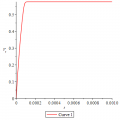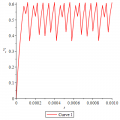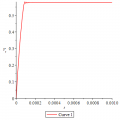Now, an even more realistic diode model that incorporates avalanche effects or breakdown effects could potentially permit oscillations, as they exhibit negative resistance (or differential negative resistance, which is similar). I have a sneaking suspicion that the equations of behavior for realistic devices like that, however, are probably even worse than the nonlinear model I originally wrote down. But in that, case it would be feasible to cause oscillations (indeed, Gunn diodes, diacs, and tunneling diodes are designed with these effects at much lower voltages than a PN junction diode or LED, so oscillations using that are a lot easier to trigger), as they are capable of negative resistance. @nsaspook has given a couple good examples of those, as well as the others in this thread.

Hopefully that clears up your question regarding the equation!

#### ApacheKid

Joined Jan 12, 2015
1,094
To answer your first question, you are correct: that's what I meant, but also that, if V_0 is a DC voltage, then no periodic forcing also will occur.

To do math markup you need to know LaTeX. You can either surround the equation markup in a  or  - the first is for an equation block, the second is for inline equations. You can also click the three dots ("Insert...") and choose the "Insert Equation" button. It's pretty neat, I think.

For your second question, using that particular equation it may be conceivable that it would permit brief oscillations, but not in the long run. The reason why is this: if you solve that equation in terms of the derivative (so have dV_c/dt on one side and everything else on the other), then there IS a location where dV_c/dt can be negative, while V_c is positive (one of the things that will cause oscillations). This transition point is found by setting the derivative to zero and solving for V_c. The solution? A terrible, terrible mess involving a trascendental function called the "Lambert W function." However, the concavity of the solutions (the second-order derivatives) have exactly the opposite sign, meaning that the the closer you get to that point as V_c increases to that point, the lower the derivative is going to be, until the derivative is zero. At this point, V_c will no longer change, and will instead just stick at a specific charge level. If you were to forcibly add a little more charge to the capacitor (increase V_c), it would spit the charge out almost immediately, dropping back to its steady-state solution. If you were to solve the equation numerically, and made your time step too large, you WOULD see oscillations, but those are only because the time step would cause the calculation to pass the stead-state point slightly. This is a stable point of equilibrium. An example (with R = 100, V_0 = 1, n = 1, and C = 1 uF) of this is shown below. The first one is found by solving using an adaptive time step method (RK45), and the second one is found by Euler's method with a time step of ~2*10^-5, and the third is with a time step of ~10^-5. As you can see, oscillations do occur, but are removed the smaller the time step you choose. Reality has, effectively, an infinitely-small time step (in theory, anyway), so you'll end up with something like the first plot.
View attachment 230061View attachment 230062View attachment 230063

Now, an even more realistic diode model that incorporates avalanche effects or breakdown effects could potentially permit oscillations, as they exhibit negative resistance (or differential negative resistance, which is similar). I have a sneaking suspicion that the equations of behavior for realistic devices like that, however, are probably even worse than the nonlinear model I originally wrote down. But in that, case it would be feasible to cause oscillations (indeed, Gunn diodes, diacs, and tunneling diodes are designed with these effects at much lower voltages than a PN junction diode or LED, so oscillations using that are a lot easier to trigger), as they are capable of negative resistance. @nsaspook has given a couple good examples of those, as well as the others in this thread.

Hopefully that clears up your question regarding the equation!
Again, very very interesting, much appreciated.